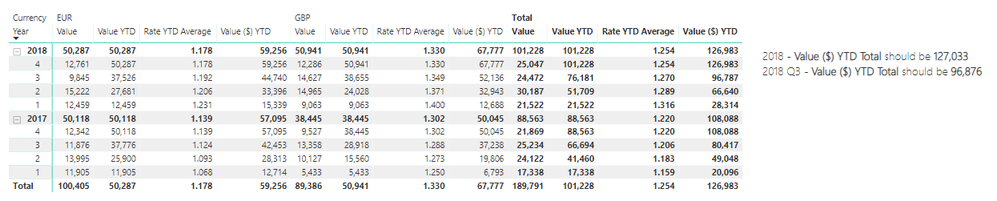cancel
Showing results for
Did you mean:Frequent Visitor

## Average Exchange Rates

Hi All,

I've posted this in the DAX Command forum as well (not sure where to place it): https://community.powerbi.com/t5/DAX-Commands-and-Tips/Average-Exchange-Rates/m-p/708507#M977

I'm having an issue trying to calculate converted values using FX rates. In the model I have a base Value in the Booking table which is in a given currency (here, GBP and EUR) (denoted by the currency column), using this value and rates I'm trying to create two measures in the base (\$) currency: Value YTD \$ and Value ITD \$ (Inception to Date).

The date dimension has months and days but the lowest granularity of time will actually be quarters. The currency conversion works as follows:

• The exchange rate is always the YTD (date range selected) average for a given currency.
• When reporting YTD Q1 2018 this should be the SUM(Q1 Values) * AVERAGE(Q1 Rates) for each currency.
• When reporting YTD Q3 2018 this should be the SUM(YTD(Q3)) * AVERAGE(YTD(Q3 Rates)) for each currency.

I have created a simple measure for this:

```Value (\$) YTD =
CALCULATE(
TOTALYTD(SUM(Booking[Value]), 'Date'[Date]) * [Rate YTD Average]
)```

However as you can see in the table below this doesn't work in row totals (it appears as if the average rate across all currencies is taken and not sub calculated per currency then totalled, this is probably a basic fix I know). I did get this working in another model correctly but honestly it looks like a hack and was brute forced.I think the above is likely easily corrected, the ITD is causing more concern as the logic for the totals are as follows:

• For inception to date reporting if the booking data starts at 2017 Q1 and ends 2018 Q4 and I filter date <= 2018 Q2 then the total shown should be SUM(YTD(2017 Q4)) * AVERAGE(YTD(2017 Q4 Rate)) + SUM(YTD(2018 Q2)) * AVERAGE(YTD(2018 Q2 Rate))

So in the table above this would essentially be a running sum from the beginning of time but applying the average rates for each year currency independently. I attempt to do just this but the context is modified by the outer SUM it seems.

Data Model:Data Model

PowerBI Template with Dummy Data and Model: Power BI Scaffold

I would greatly appreciate any help with this. I have complete control over the model, before this new exchange rate mechanism came in I was just using lookups and there was no requirement to have a relationship between the exchange table and anything else.

Best Regards,

KieranCommunity Support

You may check if the links below help.

Community Support Team _ Sam Zha
If this post helps, then please consider Accept it as the solution to help the other members find it more quickly.